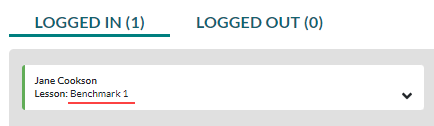# Benchmark FAQs in Imagine Math 3+

Caution

This article does not apply to students taking the NWEA™ MAP® Growth Assessment.

## 1. How many Benchmark tests are there in Imagine Math?

There are three Benchmarks. Imagine Math provides the initial Benchmark test (Benchmark 1) automatically when students first log in to their account. Imagine Math also provides two subsequent Benchmark tests (Benchmarks 2 and 3) throughout the school year which must be assigned by a teacher or administrator. Benchmarks 2 and 3 are not required but are strongly encouraged so that you can measure students' growth throughout the year.

## 2. Can the initial Benchmark be disabled?

No. Imagine Math requires that all students complete Benchmark 1 to determine the level of precursory remediation a student may need. Benchmark 1 also determines baseline data for each student.

## 3. How long are the Benchmark assessments?

Each of the three Benchmarks has been constructed to be taken within a single class sitting. Each test contains 30 multiple choice questions. Generally, students should take between 30 and 45 minutes to complete an assessment.

## 4. How can I tell who is currently taking the Benchmark?

Students taking the Imagine Math 3+ Benchmark display Benchmark as their current lesson in the Activity report.## 5. Is the Benchmark test the same for all students?

No. Imagine Math's Benchmark test questions are initially based on the student's nominal (rostered) grade level and whether they are taking Benchmark 1, 2, or 3. The Benchmark tests then adapt in difficulty in accordance with the student's responses on the test items.

## 6. Can a student pause the Benchmark test?

If students do not complete the Benchmark within a class sitting, the next time students log in to Imagine Math, they will automatically return to the Benchmark test to complete it. Once students begin a Benchmark, they are required to complete the test in its entirety before working on lessons in Imagine Math. Test progress is preserved, so students will resume the Benchmark where they left off.

For those students who do not complete the Benchmark test in a single class sitting, we recommend that you discourage them from using Imagine Math at home until the next class sitting, or until such time when you can provide proper oversight for completing the test.

## 7. What happens if a student is absent on the day of the Benchmark test?

Students who are absent on the day of the Benchmark can take the Benchmark at a later date. Since Benchmark 1 is automatically enabled, students will receive the test the first time they log in after their absence. If students are absent the day of Benchmark 2 or 3, simply do not assign them the Benchmark test in the Benchmark Roster, or, if you have already assigned it, you can unassign it and then reassign it when the students return. This ensures that students take the Benchmark under your supervision when they have returned to class.

## 8. What data does the Benchmark test generate?

Imagine Math Benchmarks determine a Quantile­® measure and a Performance Level for each student. The Quantile measure is an objective measure of each student’s abilities and readiness to learn new math skills and concepts. The Performance Level is a proficiency classification that helps you estimate how each student would perform if they took the state-level test around the same time they completed the Benchmark.

The Benchmarks also provide a Quantile Percentile Rank and a Normal Curve Equivalent (NCE) Rank for each student. All Benchmark data is available in the Benchmark report

## 9. What does "EM" stand for in a Quantile measure?

EM stands for Emerging Mathematician. Quantile measures that fall below 0 Quantile (0Q) are reported as EM---Q (e.g., a Quantile measure of -120 is reported as EM120Q) where “EM” stands for “Emerging Mathematician” and replaces the negative sign in the number. This code is predominantly seen for material and student measures at the early grade levels.